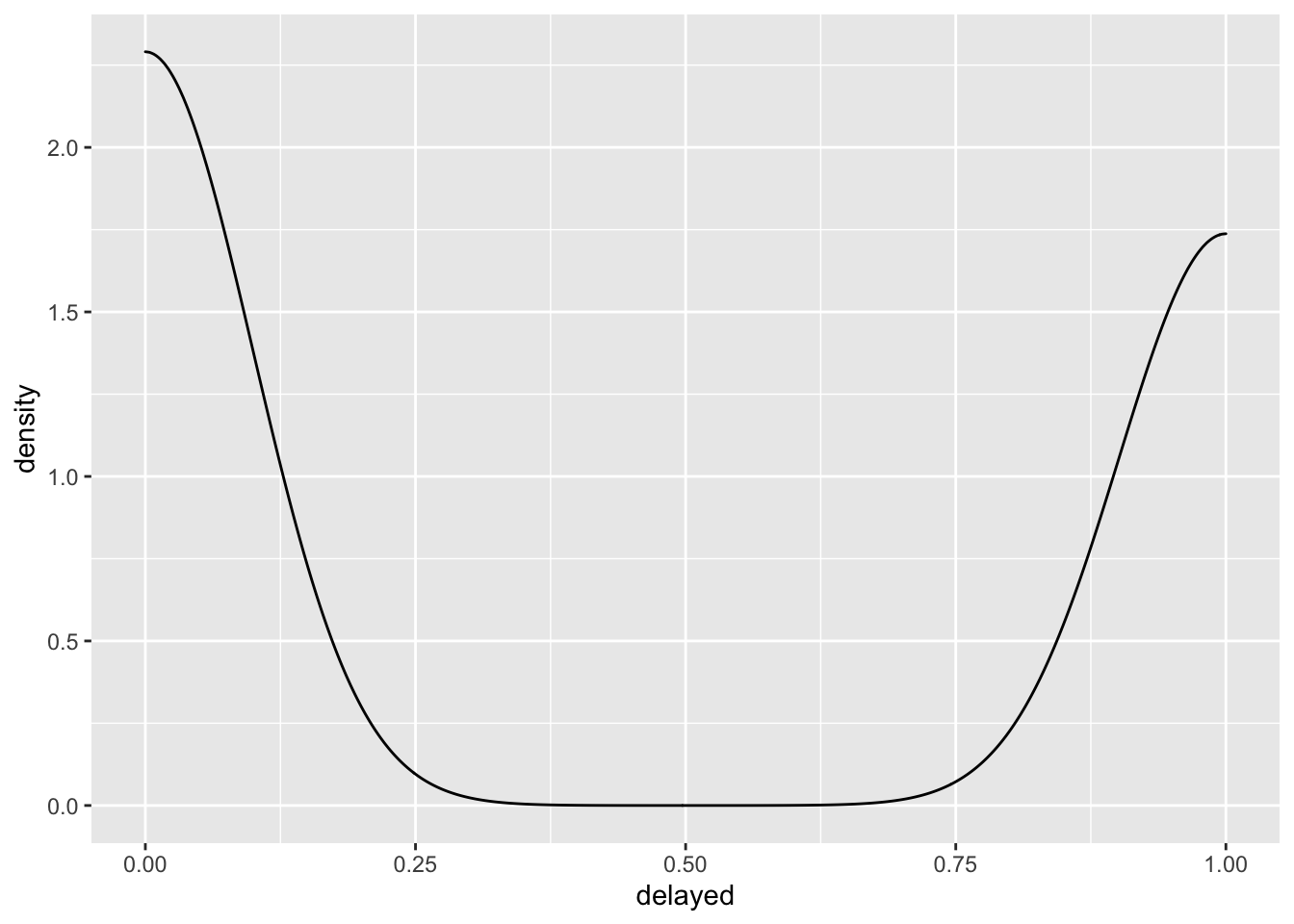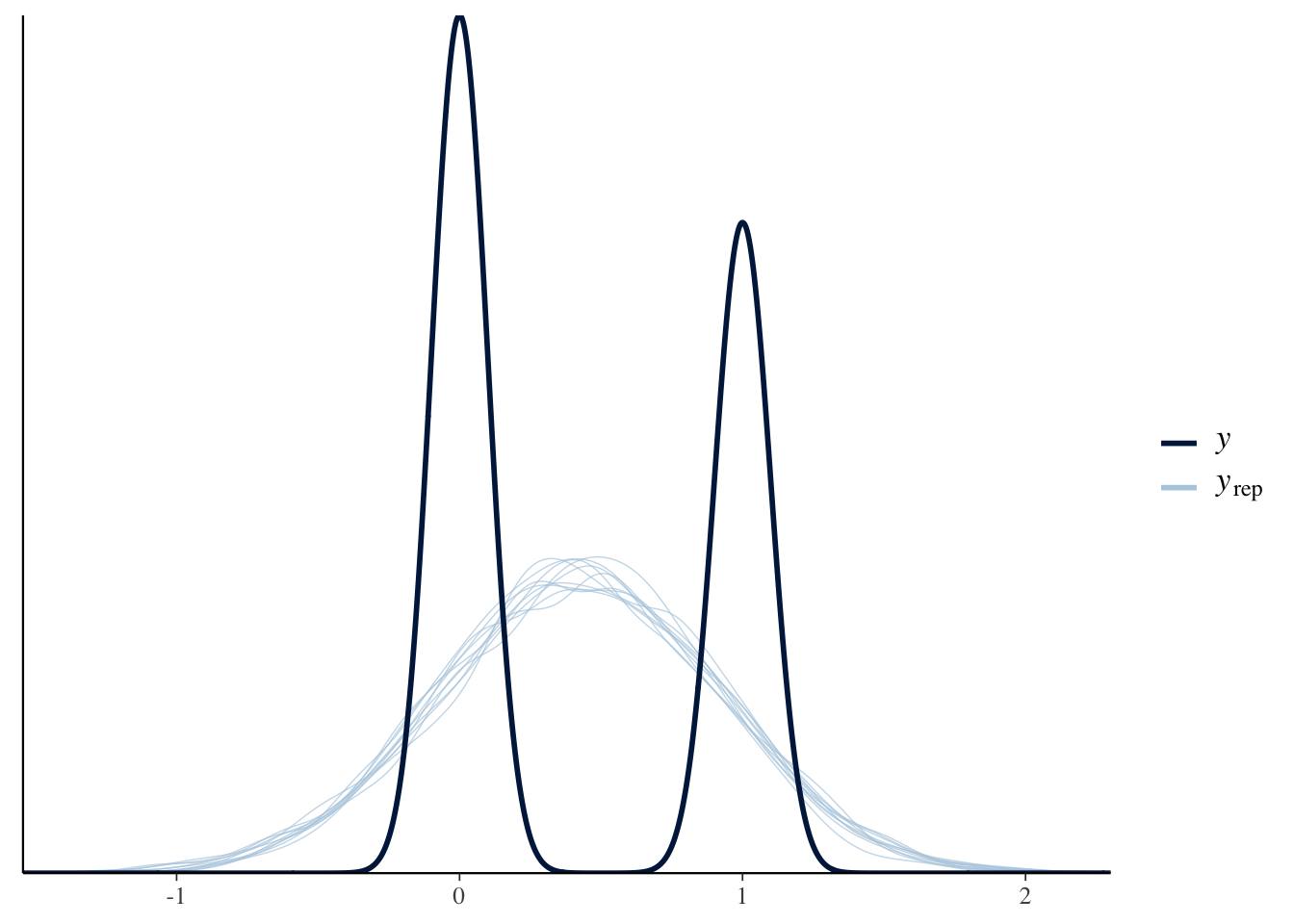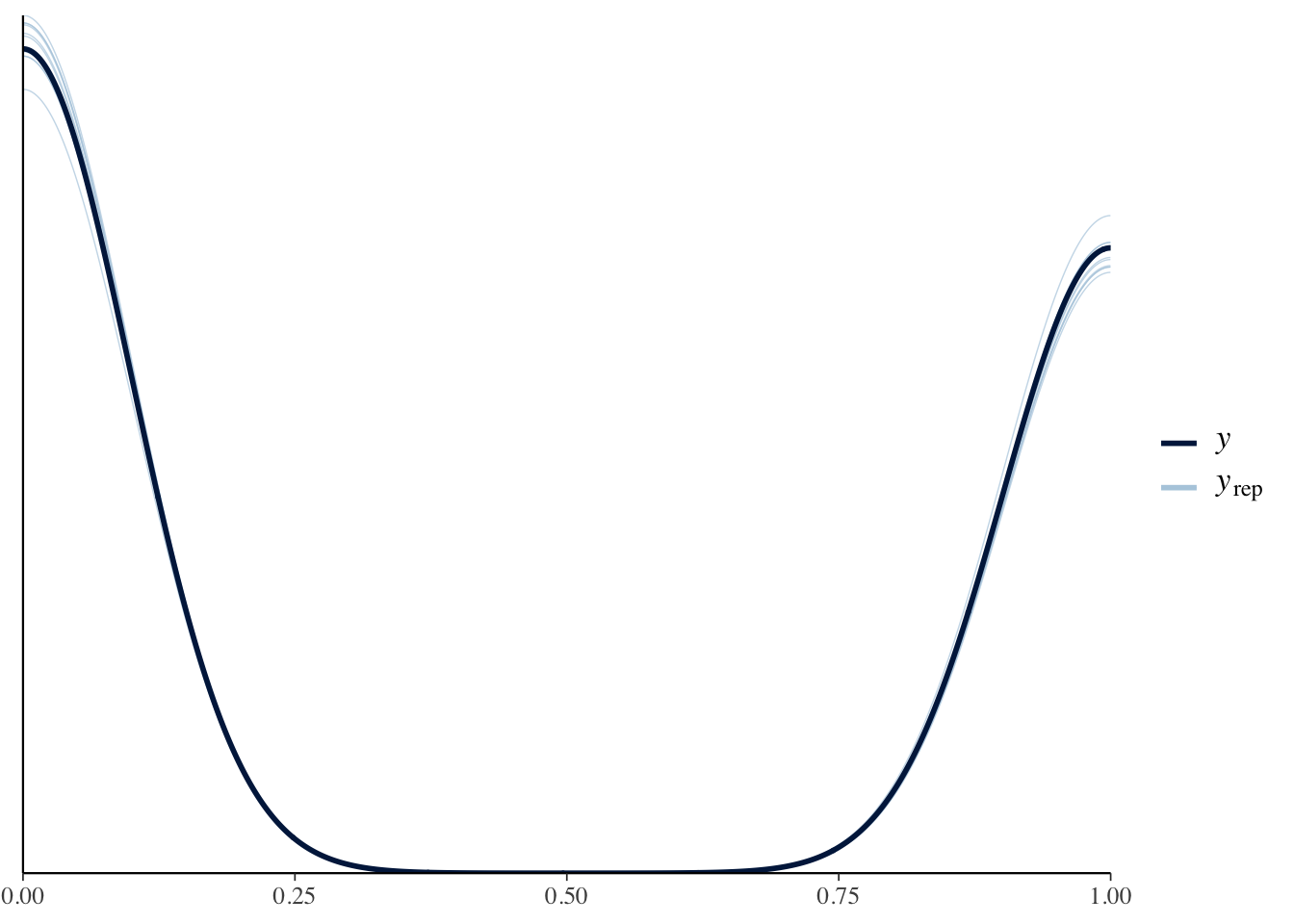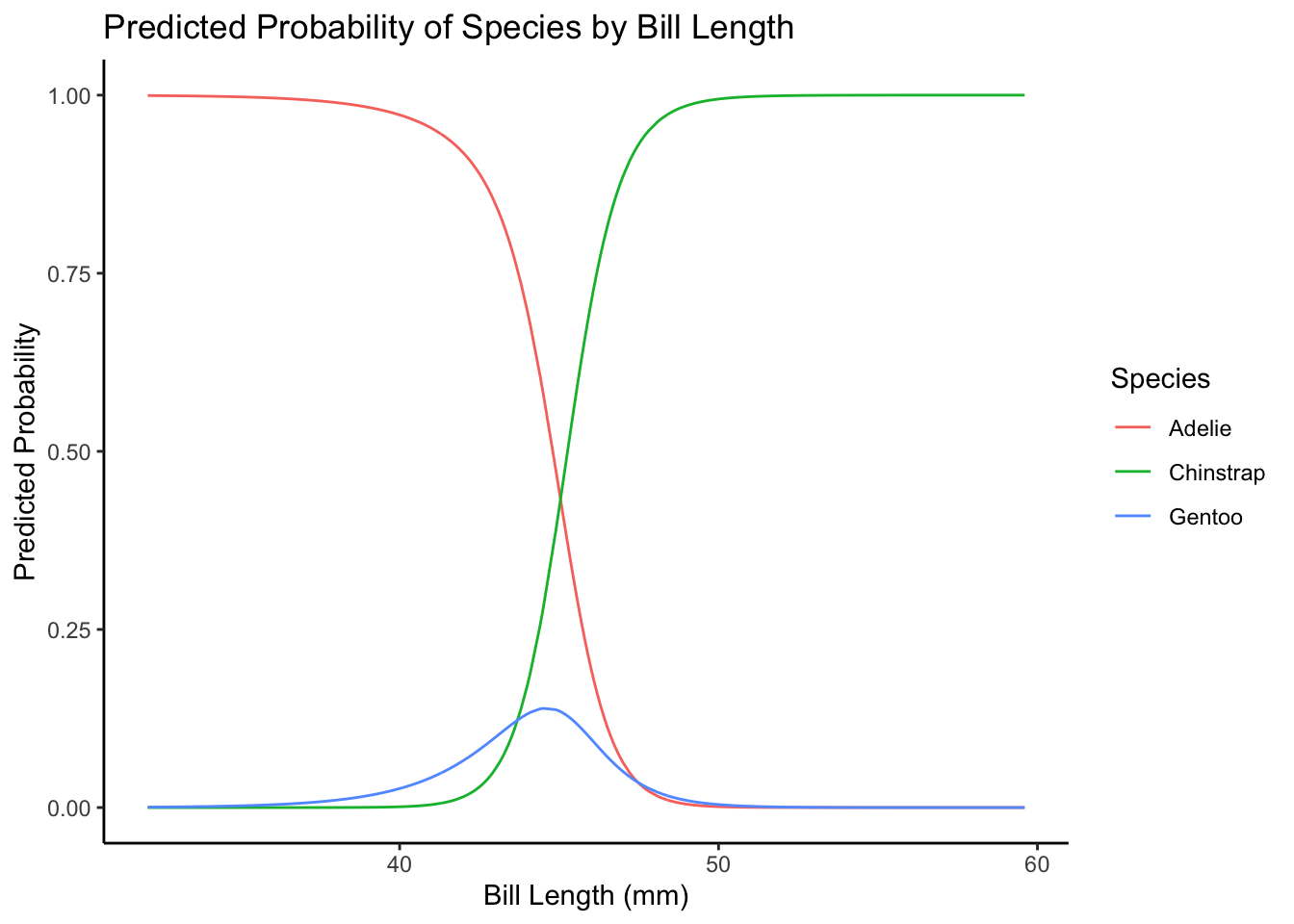# A General Guide to Generalized Linear Models

This post will walk you through how to use some of the most common generalized linear model and explain what problems they help solve and why. Before going through this code, I suggest that you take some time to review the presentation slides that I put together here.

# GLM Overview

## Forget generalizing it, what is the general linear model?

The general linear model is just the same basic linear regression model you know and love:

$y_i = \alpha + \beta x_i + \epsilon_i$

This model makes a lot of assumptions about your data, but you can’t tell that from the way that the model is written. To see what we’re hiding, let’s write this model a different way:

\begin{aligned} y_i &\sim \text{Normal}(\mu_i, \sigma^2) \\ \mu_i &= \alpha + \beta \times x_i \end{aligned}

where $$\sim$$ means “distributed”. So, $$y$$ is normally distributed with a mean of $$\mu$$ and a constant variance of $$\sigma^2$$. Since we want to estimate the average outcome, we write the model as a model of the mean with some linear equation: $$\mu = \alpha + \beta * x$$.

So,

\begin{aligned} y_i &\sim \text{Normal}(\mu_i, \sigma^2) \\ \mu_i &= \alpha + \beta \times x_i \end{aligned}

is the more complete way to express:

$y = \alpha + \beta x_i + \epsilon_i$

because it tells us what likelihood and link (more on this later) functions we are using.

### What are likelihood functions, again?

Likelihood functions are essentially probability models of our data. They provide a mathematical model of the process that generates our data. Remember, the goal of statistical modeling is to build models that can make accurate predictions and describe data! We do this by choosing likelihood functions that describe observed data.

## Generalizing the General Linear Model

### What if the normal distribution doesn’t describe our data?

As an example, we’re going to use data on flights in the US:

head(flights)
## # A tibble: 6 × 23
##    year month   day dep_time sched_dep_time dep_delay arr_time sched_arr_time
##   <int> <int> <int>    <int>          <int>     <dbl>    <int>          <int>
## 1  2013    12     3     1809           1815        -6     2051           2127
## 2  2013     9    27     1516           1520        -4     1739           1740
## 3  2013     5     2     1350           1330        20     1645           1622
## 4  2013     9    21     1434           1420        14     1555           1553
## 5  2013     8    23     1939           1830        69     2238           2126
## 6  2013    10     4     1900           1900         0     2248           2235
## # … with 15 more variables: arr_delay <dbl>, carrier <chr>, flight <int>,
## #   tailnum <chr>, origin <chr>, dest <chr>, air_time <dbl>, distance <dbl>,
## #   hour <dbl>, minute <dbl>, time_hour <dttm>, flight_delayed <chr>,
## #   delayed <dbl>, distance_c <dbl>, distance_2sd <dbl>

Let’s try to see if the distance of a flight can predict whether the flight is delayed or not. What probability model would describe flight delays? The normal distribution?

ggplot(flights, aes(x = delayed)) +
geom_density()The normal distribution doesn’t look right to me, not to mention the fact that the normal distribution describes a data generating process that produces continuous values from $$-\infty$$ to $$\infty$$. Let’s run with it anyway

normal_fit <- glm(delayed ~ distance_2sd,
family = gaussian(),
data = flights)
library(bayesplot)
pp_check(normal_fit)The normal distribution doesn’t model the data very well. How can we tell? Well, in the plot about we see the distribution of the outcome in the darker line labeled $$y$$ and simulated draws from the model in the light blue lines labeled $$y_{rep}$$. If our model was capturing the data generating process, the simulated draws $$y_{rep}$$ would match the actual data $$y$$.

What we need is a likelihood function that describes a data generating process that produces discrete values of either 0 or 1. That is exactly what the binomial and Bernoulli distributions describe. Let’s write out that model:

\begin{aligned} y_i &\sim \text{Binomial}(1, p_i) \\ \text{logit}(p_i) &= \alpha + \beta \times x_i \end{aligned}

This formulation shows the likelihood and link functions.

## Connecting the Dots

Our models always use the same linear structure ($$\mu_i = \alpha + \beta x_i$$) but, we can use any likelihood and link function that we want. With these two pieces, we can generalize our linear equation to model any type of data. Hence, the generalized linear model. It always has a linear equation, but it doesn’t always have a normal distribution!

Let’s go back to modeling flight delays, but this time with a more realistic likelihood function - the binomial.

\begin{aligned} y_i &\sim \text{Binomial}(1, p_i) \\ \text{logit}(p_i) &= \alpha + \beta \times x_i \end{aligned}

binomial_fit <- glm(delayed ~ distance_2sd,
data = flights)
pp_check(binomial_bfit)Here, the simulations from the model match the distribution of the actual data MUCH better.

Ok, let’s get into the code with some hands-on practice.

# Environment Prep

Before we get started, we need to load the tidyverse package. We’ll also load broom for tidy summaries of our model results.

library(tidyverse)
library(broom)

# Modeling Titanic Survival Data

Let’s start learning how to use GLMs with data on Titanic survival rates. This dataset is available in R and is called Titanic. Let’s get it loaded:

data("Titanic")

This code loads the data as an array, but we can convert it to a data frame like this:

titanic_data <- as.data.frame(Titanic)

We want to know if the passenger class predicts an individual’s survival rate. So, our predictor of interest is Class and the outcome is an indicator variable (0-1) for whether or not an individual survived. What kind of model should we use? We have a data generation process that produces discrete positive values that are either 0 or 1. So, we have two questions to answer:

1. What likelihood should we use?
2. What link function should we use?

The binomial likelihood function is a good choice in this case because the binomial distribution is a distribution of discrete values of either 0 or 1. But how do we map the linear predictor to the range of 0 and 1? For that we need a logit link function. Let’s see how to implement these.

## Binomial Regression

### Binomial Exercises

Now you practice. Run the code block below to disaggregate the Titanic data:

titanic_data_2 <-
titanic_data %>%
mutate(New_Response = map(Freq, ~ rep_len(1, .x))) %>%
unnest(cols = c(New_Response)) %>%
select(-Freq, -New_Response)

With the data disaggregated, try answering the following questions:

1. Run a standard logistic regression model with the disaggregated data (binomial likelihood function, logit link function).

2. Run a probit regression with the disaggregated data (binomial likelihood function, probit link function).

# Modeling Airport Passenger Traffic

In this example, we are going to build a model of airport passengers to see if the COVID lock downs imposed in March of 2020 affected the number of travelers. Let’s load in the data and do some prep.

# load the data
tsa_data <- read_csv("https://raw.githubusercontent.com/mikelor/TsaThroughput/main/data/processed/tsa/throughput/TsaThroughput.LAX.csv")
## Rows: 27551 Columns: 15
## ── Column specification ────────────────────────────────────────────────────────
## Delimiter: ","
## dbl  (13): LAX Suites, LAX TBIT Main Checkpoint, LAX Terminal 1 - Passenger,...
## date  (1): Date
## time  (1): Hour
##
## ℹ Use spec() to retrieve the full column specification for this data.
## ℹ Specify the column types or set show_col_types = FALSE to quiet this message.
#
#install.packages("janitor")
#install.packages("lubridate")
tsa_data <-
tsa_data %>%
# fix the variable names
janitor::clean_names() %>%
# create an indicator for March 2020
mutate(month = lubridate::month(date),
year = lubridate::year(date),
month_year = str_c(month, year, sep = "-"),
month_year = lubridate::my(month_year),
lockdown = ifelse(month_year == "2020-03-01", 1, 0))

How should we model this data? We want to know if the COVID lock down in March of 2020 had an effect on the number of air travelers. So, our data generating process will be positive between $$0$$ and $$-\infty$$ (theoretically) and discrete.

1. What distribution describes a process like this?
2. What link function should we use?

For these data, the Poisson distribution is a good choice for a likelihood since it describes a discrete process that generates a count of events taking place. This means that the Poisson distribution is defined for values greater than $$0$$ all the way to $$-\infty$$. In other words, it works for all positive values. Technically, the Poisson distribution is undefined for values of 0. So if we had a data generating process that produced positive values including $$0$$, we would need to use a zero-inflated Poisson likelihood function.

Now that we know what likelihood to use, how do we map the linear predictor to the range of all positive values? We’ll use the log link function.

## Poisson Regression

Now we’re ready to model passenger traffic. The outcome will be lax_tbit_main_checkpoint and the predictor will be lockdown. We’ll use a Poisson likelihood function and a log link function. Mathematically, we write this model as:

\begin{aligned} y_i &\sim \text{Poisson}(\lambda_i) \\ \text{log}(\lambda_i) &= \alpha + \beta \times x \end{aligned}

poisson_fit1 <- glm(lax_tbit_main_checkpoint ~ lockdown,
data = tsa_data)
tidy(poisson_fit1, conf.int = TRUE)
## # A tibble: 2 × 7
##   term        estimate std.error statistic p.value conf.low conf.high
##   <chr>          <dbl>     <dbl>     <dbl>   <dbl>    <dbl>     <dbl>
## 1 (Intercept)    6.44   0.000260    24772.       0    6.44      6.44
## 2 lockdown      -0.220  0.00181      -121.       0   -0.223    -0.216

### Doesn’t everyone use Negative-Binomial instead of Poisson?

Usually, where we see someone using a count model, they use a negative binomial regression instead of a Poisson regression. Well, it turns out that the negative Binomial model doesn’t involve a Binomial distribution. It’s much more accurate to call it a Gamma-Poisson model. Why Gamma-Poisson and why do we use it? One limitation of the Poisson distribution is that it assumes that the mean is equal to the variance. When this isn’t the case, we have either over, or under, dispersion. This is where the Gamma-Poisson model helps and we can write it like this:

\begin{aligned} y_i &\sim \text{Gamma-Poisson}(\lambda_i, \phi) \\ \text{log}(\lambda_i) &= \alpha + \beta \times x \\ \phi &\sim \text{Gamma}(0.01, 0.01) \end{aligned}

Instead of assuming the mean and variance are equal, the Gamma-Poisson model has an additional parameter ($$\phi$$) that allows each observation to have its own rate (it lets the mean differ from the variance). And, the rate parameter follows a gamma distribution because the gamma distribution is a positive continuous distribution defined between (0 and $$\infty$$). Let’s run it.

#install.packages("MASS")
library(MASS)
##
## Attaching package: 'MASS'
## The following object is masked from 'package:dplyr':
##
##     select
gp_fit1 <- glm.nb(lax_tbit_main_checkpoint ~ lockdown,
data = tsa_data)

tidy(gp_fit1, conf.int = TRUE)
## # A tibble: 2 × 7
##   term        estimate std.error statistic     p.value conf.low conf.high
##   <chr>          <dbl>     <dbl>     <dbl>       <dbl>    <dbl>     <dbl>
## 1 (Intercept)    6.44    0.00685    941.   0              6.43      6.45
## 2 lockdown      -0.220   0.0428      -5.13 0.000000288   -0.302    -0.135

Because the Gamma-Poisson model involves two likelihood functions, it is considered a mixture model. Mixture models are models that combine two likelihood functions in order to describe a data generating process.

So, how do we interpret this model?

(1 - exp(coef(gp_fit1)[])) * 100
##  19.72062

Air travel decreased by about 20 in March of 2020.

# Modeling Penguin Species

For the next example, we will build a multinomial logistic regression to classify penguin species. This data comes from the palmerpenguins R package. Let’s load it in.

#install.packages("palmerpenguins")
library(palmerpenguins)

data(penguins)

Since we want to model penguin species, let’s figure out how many types of species we have in the data:

flipper_length_mm = mean(penguins$flipper_length_mm, na.rm = T)) %>% drop_na() plot_data <- cbind(pred_data, predict(multinom_fit, newdata = pred_data, type = "probs")) plot_data <- plot_data %>% pivot_longer(cols = c(Adelie, Chinstrap, Gentoo), names_to = "species", values_to = "probs") ggplot(data = plot_data, aes(x = bill_length_mm, y = probs, color = species)) + geom_line() + labs(x = "Bill Length (mm)", y = "Predicted Probability", color = "Species", title = "Predicted Probability of Species by Bill Length") + theme_classic()# Bonus: Modeling Proportions Sometimes we want to build a model that can make predictions about proportions. Proportions are a new type of data because they are continuous and limited between 0 and 1. As an example of this, we’ll use some data on gasoline yields from crude oil as a function of covariates. #install.packages("betareg") library(betareg) data("GasolineYield") glimpse(GasolineYield) ## Rows: 32 ## Columns: 6 ##$ yield    <dbl> 0.122, 0.223, 0.347, 0.457, 0.080, 0.131, 0.266, 0.074, 0.182…
## $gravity <dbl> 50.8, 50.8, 50.8, 50.8, 40.8, 40.8, 40.8, 40.0, 40.0, 40.0, 3… ##$ pressure <dbl> 8.6, 8.6, 8.6, 8.6, 3.5, 3.5, 3.5, 6.1, 6.1, 6.1, 6.1, 6.1, 6…
## $temp10 <dbl> 190, 190, 190, 190, 210, 210, 210, 217, 217, 217, 220, 220, 2… ##$ temp     <dbl> 205, 275, 345, 407, 218, 273, 347, 212, 272, 340, 235, 300, 3…
## \$ batch    <fct> 1, 1, 1, 1, 2, 2, 2, 3, 3, 3, 4, 4, 4, 4, 5, 5, 5, 6, 6, 6, 7…

Here the yield column shows the proportion of crude oil converted to gasoline after distillation and fractionation. This will be our outcome variable. But how should we model it? We need a probability distribution that describes a data generating process that produces continuous values bounded between 0 and 1. Introducing the beta distribution!

## Beta Regression

The beta distribution fits the bill exactly. It’s continuous and limited between 0 and 1. But what link function? Well, we already know of a link function that restricts a linear model to be between 0 and 1 - the logit link function. Let get to it:

beta_fit <- betareg(yield ~ gravity + pressure + temp10,
data = GasolineYield)

tidy(beta_fit, conf.int = TRUE)
## # A tibble: 5 × 8
##   component term        estimate std.error statistic  p.value conf.low conf.high
##   <chr>     <chr>          <dbl>     <dbl>     <dbl>    <dbl>    <dbl>     <dbl>
## 1 mean      (Intercept) -2.98      2.80      -1.06    2.87e-1  -8.47      2.51
## 2 mean      gravity     -0.00213   0.0265    -0.0806  9.36e-1  -0.0540    0.0497
## 3 mean      pressure     0.150     0.102      1.47    1.41e-1  -0.0498    0.351
## 4 mean      temp10       0.00416   0.00789    0.528   5.98e-1  -0.0113    0.0196
## 5 precision (phi)       14.8       3.65       4.05    5.10e-5   7.63     21.9

Just like with logistic regression, we can interpret these coefficients on the probability scale by using the inverse logit (logistic) link function plogis(). So, the proportion of gasoline yielded from crude oil increases by plogis(0.15) about 54% for a one unit increase in pressure. Accounting for the intercept, it’s an absolute increase of only plogis(-2.98 + 0.15) - plogis(-2.98) 1%.##### Nick Jenkins
###### Data Scientist

Quantitative Social Scientist